## Summary

Of course everything we render so far is made of polygons, but someone asked me how to clip a polygon shape based on a list of points in a shader so I’ll explain how to do that now. I will explain how to do that with a single shader pass in a fragment shader, a different way would be to actually generate triangles based on your polygon and use stencil buffers to clip, but I won’t explain that in this tutorial.

Because this tutorial explains a simple technique that doesn’t do that much with fancy graphics I will explain it in a unlit shader, but it will work the same way in surface shaders. The base for this tutorial will be my simple shader with properties, so you should know how to do that before starting this tutorial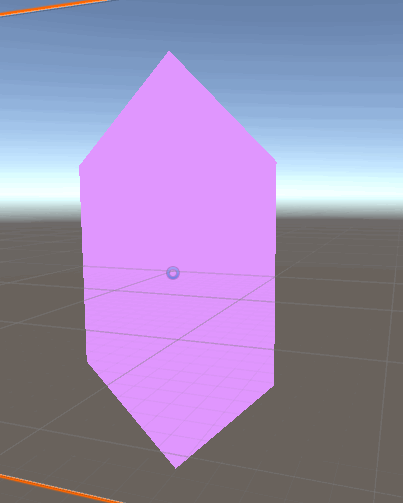## Draw Line

The first thing we have to add to our shader is the world position. Like in the other shaders (planar, triplanar and chessboard) we do that by multiplying the object position with the object to world matrix and pass that value to the fragment shader.

``````//the data that's used to generate fragments and can be read by the fragment shader
struct v2f{
float4 position : SV_POSITION;
float3 worldPos : TEXCOORD0;
};

v2f vert(appdata v){
v2f o;
//convert the vertex positions from object space to clip space so they can be rendered
o.position = UnityObjectToClipPos(v.vertex);
//calculate and assign vertex position in the world
float4 worldPos = mul(unity_ObjectToWorld, v.vertex);
o.worldPos = worldPos.xyz;
return o;
}
``````

Then we can progress to the fragment shader. here we start by calculating which side of a line a point is of. Since we will later generate our lines based on points, it’s easiest to define them as two points the line goes through.

To calculate which side of the line a point is on, we generate two vectors, first, a vector which goes from a arbitrary point of the line to our point and second the “line normal”. Usually the concept of a line normal doesn’t make much sense, but here we need a left and a right side of the line so we can define the line normal as a vector that points orthogonally to the left of the line direction.

When we have those vectors we can calculate their dot product and get the side the point is on. If the dot product is positive, the vector to the point points somewhat in the same direction as the line normal and it’s on the side the line normal points towards. If the dot product is negative the vector to the point points somewhat in the opposite direction as the line normal and the point is on th other side. If the dot product is exactly zero, the vector to the point is orthogonal to the line normal and the point is on the line.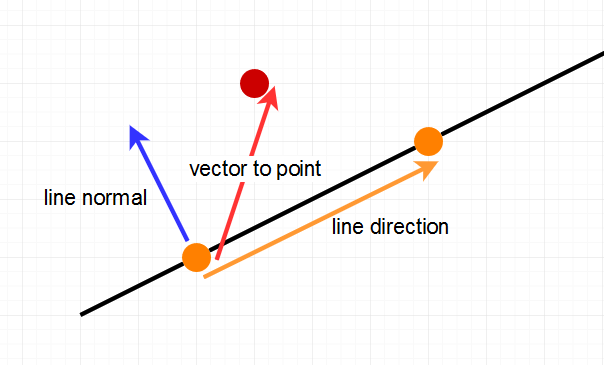To do this in shader code, we start by defining two points that define a line and then calculating those three vectors we need. We start by calculating the line direction. We get it by subtracting the first from the second line point (when calculating the difference between two points we always have to subtract the start from the goal if we care about the direction). Then we rotate the line point by 90 degree by switching it’s x and y components and inverting the new x part(if we inverted the y part we’d have a vector that points to the right of the line). And lastly we subtract one of the points defining the line from the point we’re checking to get the vector to the point.

After that we take the dot product of the line normal and the vector to the point and draw it to the screen.

``````float2 linePoint1 = float2(-1, 0);
float2 linePoint2 = float2(1, 1);

//variables we need for our calculations
float2 lineDirection = linePoint2 - linePoint1;
float2 lineNormal = float2(-lineDirection.y, lineDirection.x);
float2 toPos = i.worldPos.xy - linePoint1;

//which side the tested position is on
float side = dot(toPos, lineNormal);
side = step(0, side);

return side;
``````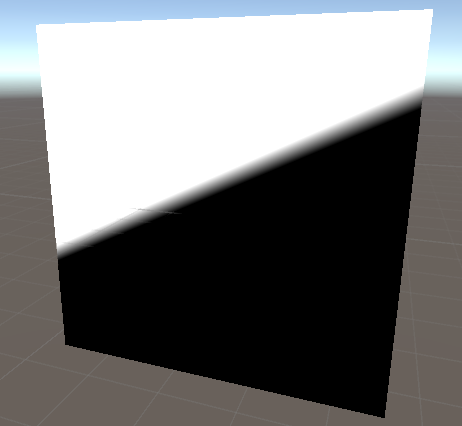As you can see, we actually see a small gradient at the line we defined. But we don’t really want a gradient, we want a clear differentiation. The gradient is here, because all colors below 0 (to the right of the line) are counted as black, all colors between 0 and 1 (just to the left of the line) are greyscale values and all colors of 1 and higher(way to the left of teh line) are displayed as white. A easy fix for that is the step function which takes two values and returns 0 if the value to the left is bigger and 1 otherwise. So if we give the step function a 0 and the result of our dor product it will give us a clear distinction between the two sides.

``````//which side the tested position is on
float side = dot(toPos, lineNormal);
side = step(0, side);

return side;
``````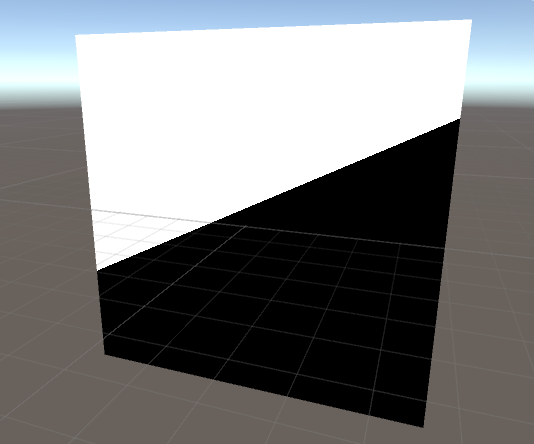We continue by adding a new point and two new lines which should allow us to make a triangle. For that it’s best to put the calculations we made so far in a method to reuse them more easily. For that we move all of our calculations to a new method and take the information we use as arguments, so in this case we want to take the point we want to check, the first point of the line and the second point of the line as arguments.

``````//return 1 if a thing is left of the line, 0 if not
float isLeftOfLine(float2 pos, float2 linePoint1, float2 linePoint2){
//variables we need for our calculations
float2 lineDirection = linePoint2 - linePoint1;
float2 lineNormal = float2(-lineDirection.y, lineDirection.x);
float2 toPos = pos - linePoint1;

//which side the tested position is on
float side = dot(toPos, lineNormal);
side = step(0, side);
return side;
}

fixed4 frag(v2f i) : SV_TARGET{
float2 linePoint1 = float2(-1, 0);
float2 linePoint2 = float2(1, 1);

side = isLeftOfLine(i.worldPos.xy, linePoint1, linePoint2);

return side;
}
``````

## Draw a Polygon of multiple lines

When we want to combine the multiple results of the lines we can do that in different ways, we can either define the result to be true if it’s to the left of all lines and false otherwise or we can say the result is true if it’s left of one or more lines and only false if it’s to the right of all lines. The triangle I defined goes clockwise, that means the left of the lines is outside, that means to differentiate between inside and outside of the polygon we have to find the union of all “left side” fragments. We do that by adding the results of the lines, the outsides will add up and have values of 1 or higher, the inside of the polygon will have a value of 0 everywhere.

``````//the fragment shader
fixed4 frag(v2f i) : SV_TARGET{
float2 linePoint1 = float2(-1, 0);
float2 linePoint2 = float2(1, 1);
float2 linePoint3 = float2(1, -1);

float outsideTriangle = isLeftOfLine(i.worldPos.xy, linePoint1, linePoint2);
outsideTriangle = outsideTriangle + isLeftOfLine(i.worldPos.xy, linePoint2, linePoint3);
outsideTriangle = outsideTriangle + isLeftOfLine(i.worldPos.xy, linePoint3, linePoint1);

return outsideTriangle;
}
``````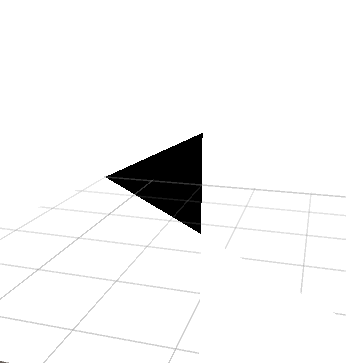Now that we can display a polygon sucessfully, I’d like to expand it so we can edit it more easily without editing the shader code. For that we add two new variables, a array of positions and how much that array is filled. The first one will hold all of the points of our polygon, the second one is there because shaders don’t support dynamic arrays, so we have to choose a length for the array and then we fill it more or less.

``````//the variables for the corners
uniform float2 _corners;
uniform uint _cornerCount;
``````

### Filling the Corner Array

There are no properties for arrays, so we have to fill them via C# code. I added two attributes to the new class, execute in edit mode to make the script update our polygon without us starting the game and require component, to make sure the script is on the same gameobject as the renderer which has the material with the shader we’re writing.

``````using System.Collections;
using System.Collections.Generic;
using UnityEngine;

[ExecuteInEditMode]
[RequireComponent(typeof(Renderer))]
public class PolygonController : MonoBehaviour {

}
``````

We then add two variables to the class, the material with the shader and a array of points which we will then pass to the shader. The material is private, because we’ll get it via code and it’s only used in this class. The position array is also private because we don’t need access from outside, but we give it the serialize field attribute to make unity remember the value and show it in the inspector.

``````[SerializeField]
private Vector2[] corners;

private Material _mat;
``````

Then we write the method which will pass the information to the shader. In it we first check if we already fetched the material and get the renderer on the gameobject and get it’s material if we didn’t. We use the sharedmaterial field of the renderer for this because if we used the material field we’d create a copy of the material which we don’t want hight here.

Then we allocate a new array of 4d vectors which can hold 1000 variables. The reason we use 4d vectors instead of the 2d vectors we need is that the unity API only allows us to pass 4d vectors and the reason for the 1000 variable length is that as I mentioned previously shaders don’t support dynamic array lengths so we have to choose a maximum of points and always choose that length, I chose 1000 pretty much randomly.

We then fill this array with the positions of our points, the 2d vectors will automatically be converted to 4d vectors with 0 at the 3rd and 4th position.

After we prepared our vector array we pass it to our material and then also pass it the amount of positions we actually use.

``````void UpdateMaterial(){
//fetch material if we haven't already
if(_mat == null)
_mat = GetComponent<Renderer>().sharedMaterial;

//allocate and fill array to pass
Vector4[] vec4Corners = new Vector4;
for(int i=0;i<corners.Length;i++){
vec4Corners[i] = corners[i];
}

//pass array to material
_mat.SetVectorArray("_corners", vec4Corners);
_mat.SetInt("_cornerCount", corners.Length);
}
``````

The next step is to actually call this function, we do this in two methods, one which we call Start and one which we call OnValidate. The first one will automatically called by unity when the game starts and the second one will automatically be called by unity when a variable of the script changes in the inspector.

``````void Start(){
UpdateMaterial();
}

void OnValidate(){
UpdateMaterial();
}
``````

After writing the script we can add it to our project to do it’s job. We just add it as a component to the same gameobject the renderer with our material is on. And when we set up our script, we can set our corners easily by adding to the array in the inspector.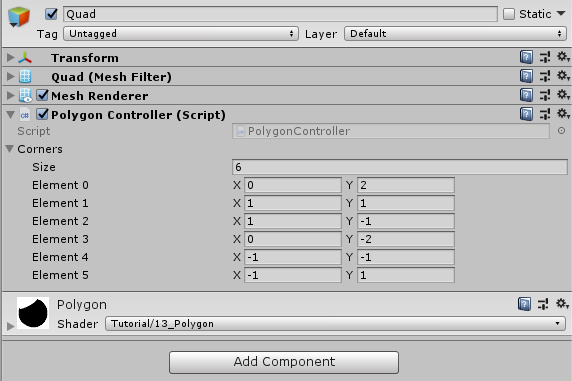Next we go back to our shader to actually use the array. To do that we instantiate our outside triangle variable as zero.

Then we iterate over the array with a typical for loop. We start the loop at 0 because the first index of arrays in hlsl is adressed 0, the second with 1 etc… we stop when the iterator value goes over the amount of corners we specified via C# and we increase the iterator by 1 every loop. We explicitely tell hlsl to loop the for loop, the alternative would be to unroll it which means it would just copypaste the stuff happening in the for loop under each other. Unrolling is usually faster in shaders, but we don’t have a fixed length in our case so we have to use loop.

In the loop, we just add the return value of the side function of one line. As the points of the line we use the corner at the position of the iterator and the corner at the position of the iterator plus one. The problem that emerges when we use that plus one is that at the last point we acess the array at a point we didn’t set, but we want to go back to the first point instead. In this position modulo helps us, we add one to the iterator and then take the modulo with the length of the valid array, that way it jumps back to 0 if it would acess a invalid value otherwise.

``````//the fragment shader
fixed4 frag(v2f i) : SV_TARGET{

float outsideTriangle = 0;

[loop]
for(uint index;index<_cornerCount;index++){
outsideTriangle += isLeftOfLine(i.worldPos.xy, _corners[index], _corners[(index+1) % _cornerCount]);
}

return outsideTriangle;
}
``````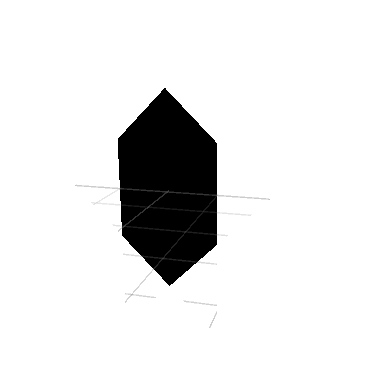And with that we have a polygon just based on a few points (if it doesn’t show for you, just nudge the values in the inspector a bit to call OnValidate).

## Clip and Color the Polygon

The person who requested this tutorial asked how to clip a polygon, so that’s the last thing we’re going to add here. In hlsl there is a function to discard polygons called clip. We pass it a value and if that value is lower than 0 the fragment won’t be rendered, otherwise the function does nothing.

We can pass the outsideTriangle variable into the clip function, but nothing will happen because all values of the value are 0 or higher. To actually clip everything outside of the polygon we can simply invert the value and the values inside of the polygon will stay 0 and all of the values outside will be negative and will be clipped.

Because we now use the outsideTriangle variable for it’s intended use, we can now stop drawing it to the screen and just print the color again.

``````    clip(-outsideTriangle);
return outsideTriangle;
}
``````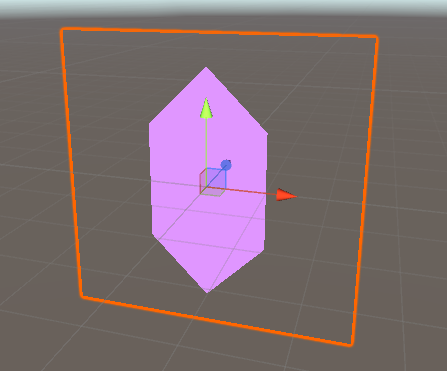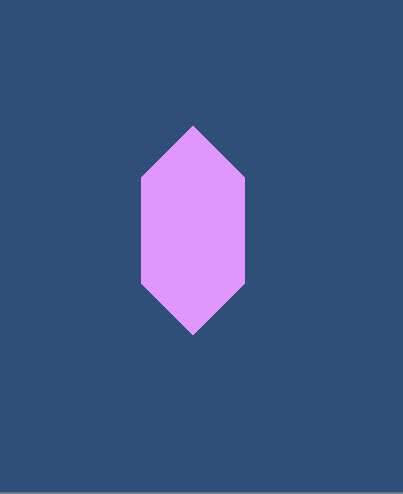The biggest disadvantage with this technique is that we can only render convex polygons, it breaks when we try to use concave ones.

``````Shader "Tutorial/014_Polygon"
{
//show values to edit in inspector
Properties{
_Color ("Color", Color) = (0, 0, 0, 1)
}

//the material is completely non-transparent and is rendered at the same time as the other opaque geometry
Tags{ "RenderType"="Opaque" "Queue"="Geometry"}

Pass{
CGPROGRAM

#include "UnityCG.cginc"

#pragma vertex vert
#pragma fragment frag

fixed4 _Color;

//the variables for the corners
uniform float2 _corners;
uniform uint _cornerCount;

//the object data that's put into the vertex shader
struct appdata{
float4 vertex : POSITION;
};

//the data that's used to generate fragments and can be read by the fragment shader
struct v2f{
float4 position : SV_POSITION;
float3 worldPos : TEXCOORD0;
};

v2f vert(appdata v){
v2f o;
//convert the vertex positions from object space to clip space so they can be rendered
o.position = UnityObjectToClipPos(v.vertex);
//calculate and assign vertex position in the world
float4 worldPos = mul(unity_ObjectToWorld, v.vertex);
o.worldPos = worldPos.xyz;
return o;
}

//return 1 if a thing is left of the line, 0 if not
float isLeftOfLine(float2 pos, float2 linePoint1, float2 linePoint2){
//variables we need for our calculations
float2 lineDirection = linePoint2 - linePoint1;
float2 lineNormal = float2(-lineDirection.y, lineDirection.x);
float2 toPos = pos - linePoint1;

//which side the tested position is on
float side = dot(toPos, lineNormal);
side = step(0, side);
return side;
}

fixed4 frag(v2f i) : SV_TARGET{

float outsideTriangle = 0;

[loop]
for(uint index;index<_cornerCount;index++){
outsideTriangle += isLeftOfLine(i.worldPos.xy, _corners[index], _corners[(index+1) % _cornerCount]);
}

clip(-outsideTriangle);
return _Color;
}

ENDCG
}
}
}
``````
``````using System.Collections;
using System.Collections.Generic;
using UnityEngine;

[ExecuteInEditMode]
[RequireComponent(typeof(Renderer))]
public class PolygonController : MonoBehaviour {
[SerializeField]
private Vector2[] corners;

private Material _mat;

void Start(){
UpdateMaterial();
}

void OnValidate(){
UpdateMaterial();
}

void UpdateMaterial(){
//fetch material if we haven't already
if(_mat == null)
_mat = GetComponent<Renderer>().sharedMaterial;

//allocate and fill array to pass
Vector4[] vec4Corners = new Vector4;
for(int i=0;i<corners.Length;i++){
vec4Corners[i] = corners[i];
}

//pass array to material
_mat.SetVectorArray("_corners", vec4Corners);
_mat.SetInt("_cornerCount", corners.Length);
}

}
``````

I hope you learned something about how to approach problems with multiple points and vectors. And I hope I talked about what you wanted to know, Alex.

You can also find the source code for this tutorial here: